## SGPLOT HEATMAP colormap labels/values size

Hi,

I am using PROC SGPLOT with HEATMAP and I would like to change the size of the colormap values.

Here is my code and the resulting plot:

``````PROC SGPLOT data=  MARTA.mydata;
heatmap x=clocktime y=myvariable / colorresponse=aaa  colorstat=mean colormodel=(bigb red) xbinsize=0.25 ;
xaxis label='Clock time' values=(9 to 18 by 1) valueattrs=(size=14pt) labelattrs=(size=14pt);
yaxis label='My variable' valueattrs=(size=14pt) labelattrs=(size=14pt);
run;
``````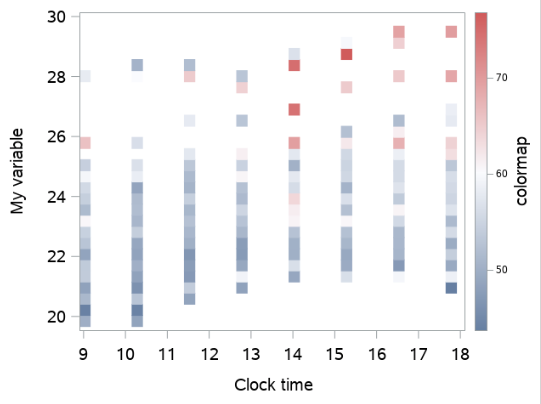My problem seems to be simple but I can not find the solution. The values of the colormap are smaller compared to the other labels and values on the axes.

How to set such values to 14pt?

1 ACCEPTED SOLUTION

Accepted Solutions

## Re: SGPLOT HEATMAP colormap labels/values size

Here's a technique that works in SAS 9.4m7 (and probably earlier versions as well), using a custom ods style to control most of the normal (non-title/non-label) text in the graph. Since I don't have your data, I use some 'random' data from the sashelp sample lib to demonstrate.

ods path(prepend) work.templat(update);
proc template;
define style my_style;
parent=styles.htmlblue;
class GraphValueText / font = ("<sans-serif>, <MTsans-serif>",14pt);
end;
run;

ods html style=my_style;

proc sgplot data=sashelp.class;
heatmap x=height y=weight / colorresponse=weight colorstat=mean colormodel=(bigb red) xbinsize=0.25 ;
xaxis label='Clock time' labelattrs=(size=14pt);
yaxis label='My variable' labelattrs=(size=14pt);
run;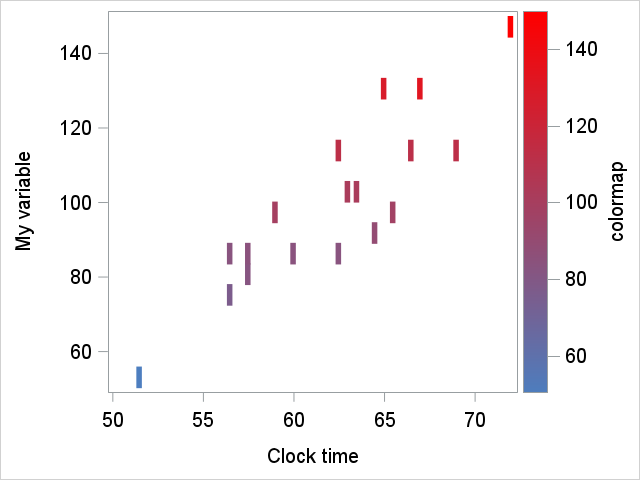15 REPLIES 15

## Re: SGPLOT HEATMAP colormap labels/values size

The attributes in the interior of the heat map do not know about the attributes for the axis labels or ticks.

What do you mean by "the values of the colormap"? Are you talking about the size of the rectangles that show the values of the aaa variable at each cell for (clocktime, myvariable)? If so, use

XBINSIZE=1

on the HEATMAP statement to have the rectangles span one unit in the x direction.  If desired, you can also use the YBINSIZE= option to change the size of the rectangles in the Y direction.

## Re: SGPLOT HEATMAP colormap labels/values size

If you mean the size of the colored heatmap areas then look at the XBINSIZE or YBINSIZE to specify a specific size. See what happens when you add Xbinsize=1 and Ybinsize=2 to the heatmap statement.

or possibly NXBINS and NYBINS (the number of bins). Try NXBINS=10 and NYBINS=10.

There are other binning options as well to examine.

Data is important as well. We do not have your data and you don't provide any clues as to "how big" or range of values you want the areas to represent.

## Re: SGPLOT HEATMAP colormap labels/values size

To get the size of the text in the legend larger, I believe you can change the size= in the valueattrs= in the gradlegend. Here's the gradlegend valueattrs= example from the doc ...

```valueattrs=(Color=Green Family=Arial Size=8
Style=Italic Weight=Bold) ```

## Re: SGPLOT HEATMAP colormap labels/values size

Hi Robert,

yes that was exactly my question and I had tried valueattrs=(size=...) as you wrote.

``gradlegend / title='colormap' titleattrs=(size=14pt) valueattrs=(size=14pt);``

My problem is that the word "valueattrs" in my code does not turn to blue: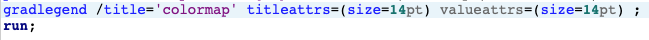...resulting in an error: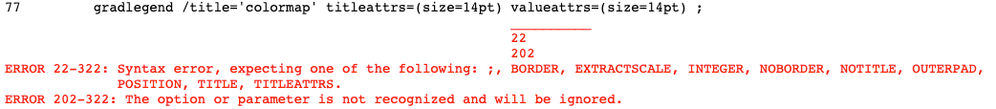I don't understand why...

## Re: SGPLOT HEATMAP colormap labels/values size

Here is the doc for the GRADLEGEND statement:

https://go.documentation.sas.com/doc/en/pgmsascdc/9.4_3.5/grstatproc/n0pejjes5ug1j2n1roobaiuj9w60.ht...

There is no VALUESATTR= option for that statement in PROC SGPLOT. If you really want this, you will have to use the GTL and the CONTINUOUSLEGEND statement: SAS Help Center: CONTINUOUSLEGEND Statement

## Re: SGPLOT HEATMAP colormap labels/values size

Oops - sorry about that! I was looking at doc for a variant/version of SAS that you probably don't have. (Hopefully this feature will make its way into the more common versions of of SAS in the near future!)

## Re: SGPLOT HEATMAP colormap labels/values size

Here's a technique that works in SAS 9.4m7 (and probably earlier versions as well), using a custom ods style to control most of the normal (non-title/non-label) text in the graph. Since I don't have your data, I use some 'random' data from the sashelp sample lib to demonstrate.

ods path(prepend) work.templat(update);
proc template;
define style my_style;
parent=styles.htmlblue;
class GraphValueText / font = ("<sans-serif>, <MTsans-serif>",14pt);
end;
run;

ods html style=my_style;

proc sgplot data=sashelp.class;
heatmap x=height y=weight / colorresponse=weight colorstat=mean colormodel=(bigb red) xbinsize=0.25 ;
xaxis label='Clock time' labelattrs=(size=14pt);
yaxis label='My variable' labelattrs=(size=14pt);
run;## Re: SGPLOT HEATMAP colormap labels/values size

It does not really work because now I get an authorization error: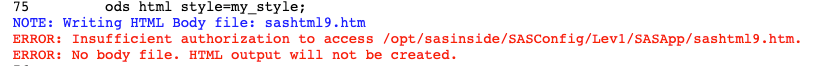## Re: SGPLOT HEATMAP colormap labels/values size

Hmm ... are you running SAS through Enterprise Guide, or SAS University Edition, or something like that?

If so, perhaps one of these threads will help:

https://communities.sas.com/t5/SAS-Enterprise-Guide/How-to-Get-HTML-output-in-EG-8-2/td-p/623073

https://communities.sas.com/t5/SAS-Analytics-U/Error-Message-in-SAS-University-Edition/td-p/425601

## Re: SGPLOT HEATMAP colormap labels/values size

Thanks, now it kind of works using this code:

``````ODS HTML path='/folders/myfolders/plots'
GPATH = '/folders/myfolders/plots/' image_dpi =300;
ods path(prepend) work.templat(update);
proc template;
define style my_style;
parent=styles.htmlblue;
class GraphValueText / font = ("<sans-serif>, <MTsans-serif>",14pt);
end;
run;
ods html path='/folders/myfolders/plots' style=my_style;
proc sgplot data=sashelp.class;
heatmap x=height y=weight / colorresponse=weight colorstat=mean colormodel=(bigb red) xbinsize=0.25 ;
xaxis label='Clock time' labelattrs=(size=14pt);
yaxis label='My variable' labelattrs=(size=14pt);
run;
``````

In this way, a .png image is saved together with a .htm file. I don't need this .htm file, is there a way to not generate it (except from deleting it manually)?

Also, can I set the name of the generated file?

I used to save plots using:

``````ods listing gpath='/folders/myfolders/plots';
ods graphics /attrpriority=none imagename="plot_heatmap"  noborder imagefmt=png;``````

...but I don't know how to set the style here.

Thanks!

## Re: SGPLOT HEATMAP colormap labels/values size

Have you tried ods graphics / imagename='plot_heatmap' to control the png name, like you were using before?

## Re: SGPLOT HEATMAP colormap labels/values size

Yes, this works to set the file name.

``````ods html path='/folders/myfolders/plots' style=my_style image_dpi=500;
ods listing gpath='/folders/myfolders/plots';
ods graphics /attrpriority=none imagename="heatmap" imagefmt=png;``````

However I still get a "sashtml.htm" file created every time I create my png plot.

Is there a way to avoid creating this .htm file?

I understand I need to keep "ods html..." to set the style...

Many thanks!

## Re: SGPLOT HEATMAP colormap labels/values size

I don't know - I've never not wanted the html file 🙂

## Re: SGPLOT HEATMAP colormap labels/values size

@marta25 wrote:

Yes, this works to set the file name.

``````ods html path='/folders/myfolders/plots' style=my_style image_dpi=500;
ods listing gpath='/folders/myfolders/plots';
ods graphics /attrpriority=none imagename="heatmap" imagefmt=png;``````

However I still get a "sashtml.htm" file created every time I create my png plot.

Is there a way to avoid creating this .htm file?

I understand I need to keep "ods html..." to set the style...

Hello @marta25,

You can send the HTML file to "nowhere" (arbitrary fileref, see documentation):

``````filename nowhere dummy;
ods html file=nowhere gpath='/folders/myfolders/plots' style=my_style image_dpi=500;``````

(I think with your additional ODS LISTING statement you also get a second PNG file, which you could avoid with ods listing close;.)

Discussion stats
• 15 replies
• 1286 views
• 9 likes
• 5 in conversation